# Samacheer Kalvi 7th Maths Solutions Term 3 Chapter 5 Statistics Ex 5.3

Students can Download Maths Chapter 5 Statistics Ex 5.3 Questions and Answers, Notes Pdf, Samacheer Kalvi 7th Maths Book Solutions Guide Pdf helps you to revise the complete Tamilnadu State Board New Syllabus and score more marks in your examinations.

## Tamilnadu Samacheer Kalvi 7th Maths Solutions Term 3 Chapter 5 Statistics Ex 5.3

Question 1.
Fill in the blanks.

(i) The median of the data 12, 14, 23, 25, 34, 11, 42, 45, 32, 22, 44 is ________
(ii) The median of first ten even natural numbers is ________
(i) 25
(ii) 11Question 2.
Find the median of the given data: 35, 25, 34, 36, 45, 18, 28.
Solution:
Arranging the given data in ascending order 18, 25, 28, 34, 35, 36, 45.
Here the number of observations n = 7, which is odd.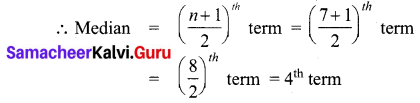Hence Median = 34

Question 3.
The weekly sale of motor bikes in a showroom for the past 14 weeks given below.
10, 6, 8, 3, 5, 6, 4, 7, 12, 13, 16, 10, 4, 7.
Find the median of the data.
Solution:
Arranging the given data in ascending order 3, 4, 4, 5, 6, 6, 7, 7, 8, 10, 10, 12, 13, 16.
Here number of data n = 14, which is even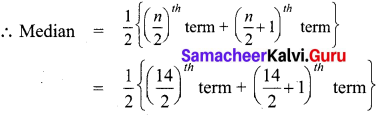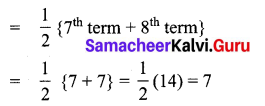∴ Median = 7Question 4.
Find the median of the 10 observations 36, 33, 45, 28, 39, 45, 54, 23, 56, 25.
If another observation 35 is added to the above data, what would be the new median?
Solution:
Arranging the given 10 observations in ascending order 23, 25, 28, 33, 36, 39, 45, 45, 54, 56.
Here number of data n = 10, which is even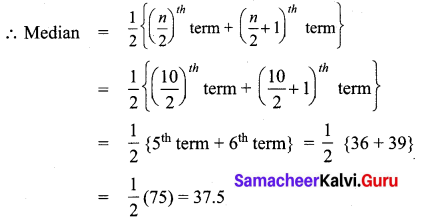∴ Median = 37.5
If 35 is added to the above data then it will be the 5th term then number of data n = 11, odd
∴ Median = ($$\frac { n+1 }{ 2 }$$)th term = ($$\frac { 11+1 }{ 2 }$$)th term
= ($$\frac { 12 }{ 2 }$$)th term = 6th term
New median = 36Objective Type Questions

Question 1.
If the median of a, 2a, 4a, 6a, 9a is 8, then find the value of a is
(i) 8
(ii) 6
(iii) 2
(iv) 10
(iii) 2
Hint: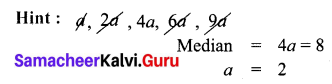Median = 4a = 8
a = 2

Question 2.
The median of the data 24, 29, 34, 38, 35 and 30, is ________
(i) 29
(ii) 30
(iii) 34
(iv) 32
(iv) 32
Hint: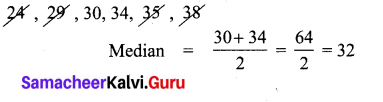Median = $$\frac { 30+34 }{ 2 }$$
= $$\frac { 64 }{ 2 }$$
= 32Question 3.
The median first 6 odd natural numbers is
(i) 6
(ii) 7
(iii) 8
(iv) 14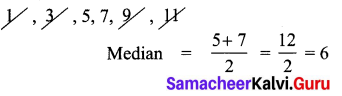Median = $$\frac { 5+7 }{ 2 }$$
= $$\frac { 12 }{ 2 }$$